# Expression for hole concentration in valence band

The hole concentration in the valence band is a measure of the number of empty states, or holes, that are available for electrons to occupy in the valence band of a material. The concentration of holes in the valence band is related to the concentration of electrons in the conduction band through the principle of charge neutrality, which states that the total charge of a material must be balanced.

In a semiconductor, the concentration of holes in the valence band is determined by the temperature and the doping level of the material. At low temperatures, most of the electrons are in the valence band, and there are very few holes. As the temperature increases, some of the electrons in the valence band gain enough energy to jump to the conduction band, leaving behind holes in the valence band. The concentration of holes in the valence band therefore increases with temperature.

We have distribution function for hole in valence band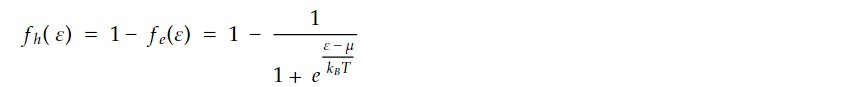Using the case µ - ε <<KBTWe have density of state for hole is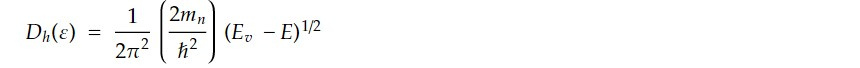Hole concentration in valance band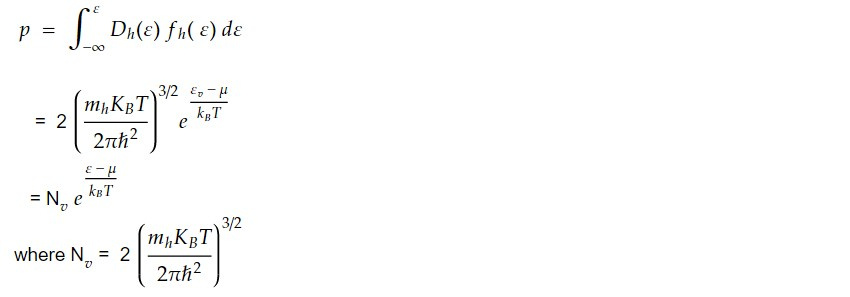This gives the hole concentration in valence band for intrinsic semi conductor.

The product of n and p is given by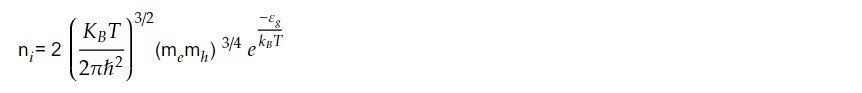This shows that the product np is always constant at a given temperature and is independent of chemical potential. This relation gives the relative concentration holes of electron in a given semiconductor.

For intrinsic semiconductor

n=p=niThis gives the intrinsic carrier concentration for a given semiconductor. We can find the band gap of the material using this relation.

For Ge, at room temperature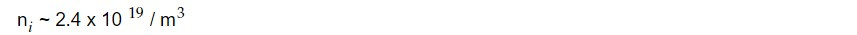Concentration of Ge atom is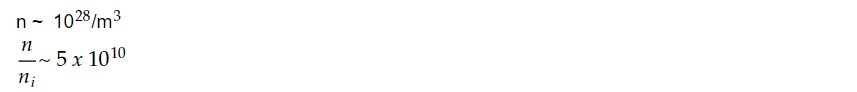At room temperature only 5 valence band is broken per 10^10 atom.

Expression for band gap

For intrinsic semiconductor n=p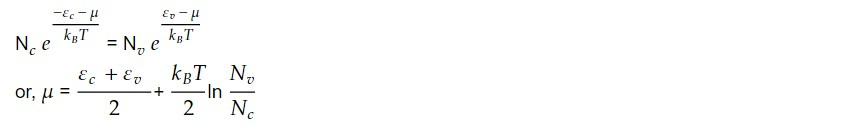This gives the expression for chemical potential for intrinsic semiconductor.

If fermi level is measured from the top of valence bond, then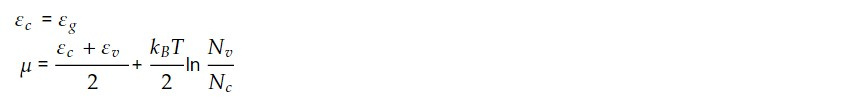Using relation for Nv and Nc we can get the relation for Band gap in terms of mobility i.e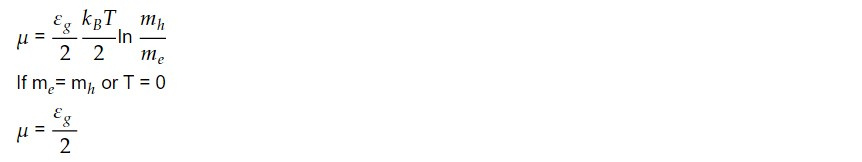Fermi level lies at the middle of gap.

The hole concentration in the valence band is an important parameter in semiconductor physics and device engineering, as it affects the electrical and optical properties of the material.

This note is a part of the Physics Repository.